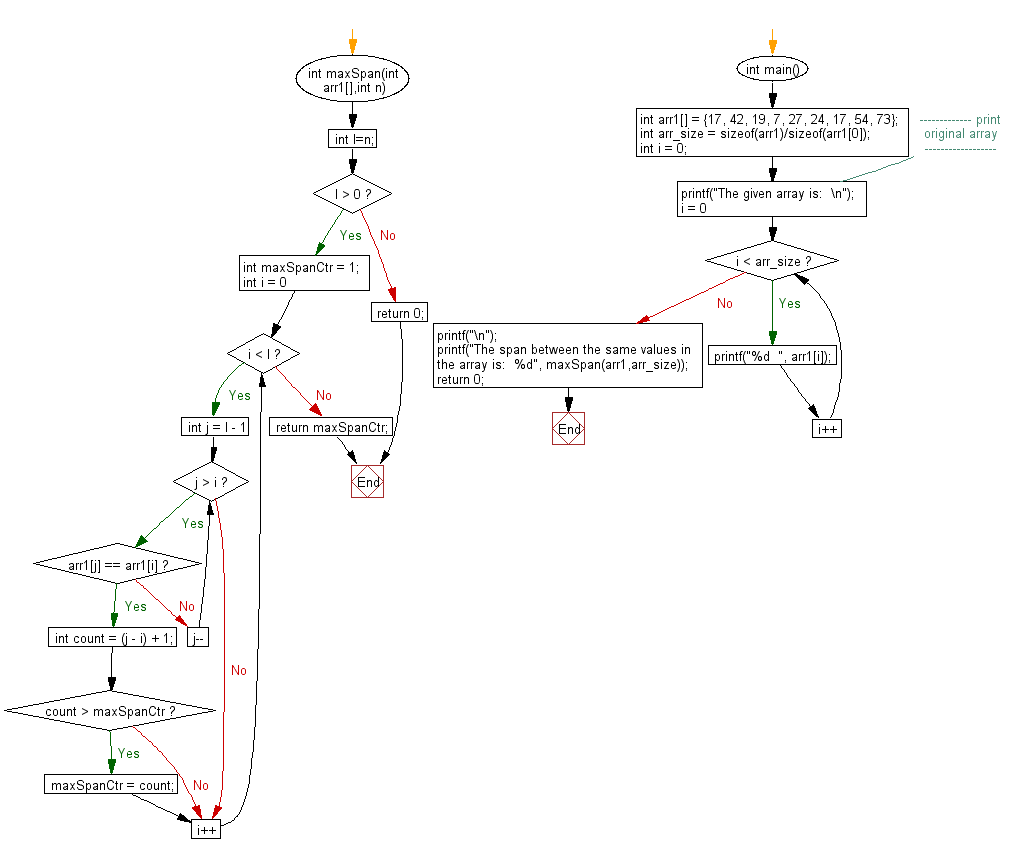﻿ C exercises: Return the largest span found in the leftmost and rightmost appearances of same value in a given array - w3resource# C Exercises: Return the largest span found in the leftmost and rightmost appearances of same value in a given array

## C Array: Exercise-98 with Solution

Write a program in C to return the largest span found in the leftmost and rightmost appearances of same value(values are inclusive) in a given array.

Sample Solution:

C Code:

``````#include<stdio.h>

int maxSpan(int arr1[],int n)
{
int l=n;
if (l > 0)
{
int maxSpanCtr = 1;
for (int i = 0; i < l; i++)
for (int j = l - 1; j > i; j--)
if (arr1[j] == arr1[i])
{
int count = (j - i) + 1;
if (count > maxSpanCtr) maxSpanCtr = count;
break;
}
return maxSpanCtr;
}
else return 0;
}
int main()
{
int arr1[] = {17, 42, 19, 7, 27, 24, 17, 54, 73};
int arr_size = sizeof(arr1)/sizeof(arr1);
int i = 0;
//------------- print original array ------------------
printf("The given array is:  \n");
for(i = 0; i < arr_size; i++)
{
printf("%d  ", arr1[i]);
}
printf("\n");
//-----------------------------------------------------------
printf("The span between the same values in the array is:  %d", maxSpan(arr1,arr_size));
return 0;
}
```
```

Sample Output:

```The given array is:
17  42  19  7  27  24  17  54  73
The span between the same values in the array is:  7
```

Pictorial Presentation:Flowchart:C Programming Code Editor:

Improve this sample solution and post your code through Disqus.

﻿